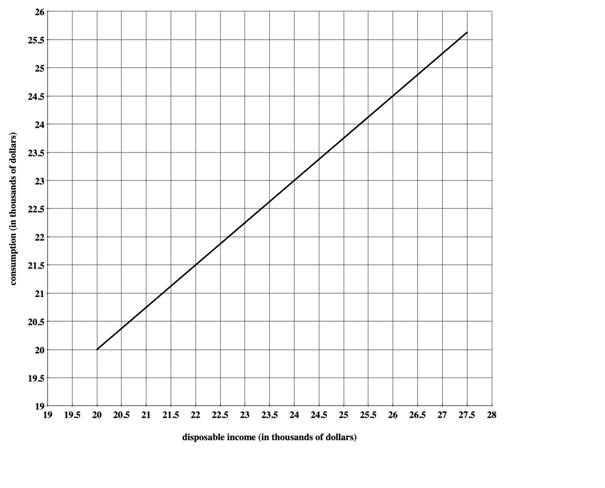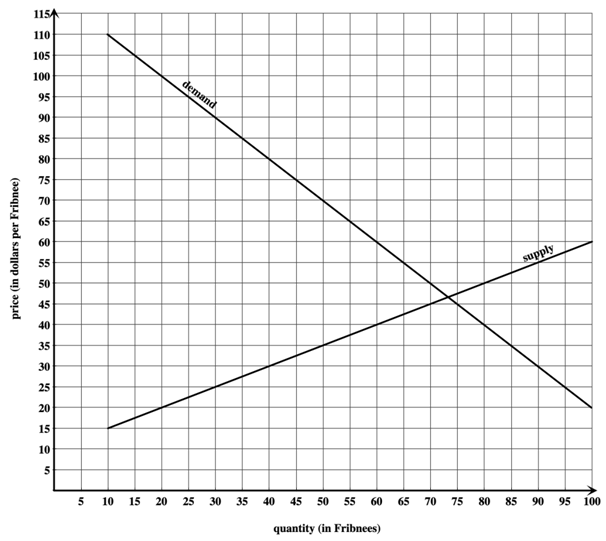## MATH 111 | Assignment Help | University of Washington

MATH 111  | Assignment Help | University of Washington

Activity 5

Question 1.

Suppose a consumer's consumption y, the amount of money spent in a specified time period, is related to their disposable income x earned in that time period by the linear function shown below.  In functional notation, y=f(x), where f is a linear function of xUse the graph to approximate the consumption for a consumer with disposable income of 25.5 thousand dollars (\$25500).  Give your answer in thousands of dollars.

ANSWER:  _________ thousand dollars

Question 2

Using the graph in Question 1, estimate the disposable income of a consumer whose consumption is 21.5 thousand dollars.  Give your answer in thousands of dollars.

ANSWER:  _________ thousand dollars

Do not include units in the answer box.  Just a number.

Question 3

Using the graph in Question 1, find two "nice" points on the graph of the line and use them to find the slope.

ANSWER:  m=_________

Do not include units in the answer box.  Just a number.  Express your answer as a decimal.

Question 4

Use one of the "nice" points and the slope you found in the previous question to find b, the y-intercept of the line.

ANSWER:  b=________

Do not include units in the answer box. Just a number. Express your answer as a decimal.

You should now have a linear formula for the line in the form y=mx+b, where m is the slope and b is the y-intercept of the line.

Question 5

Use the linear formula you found for the line to compute the value of consumption for a consumer with 23.5 thousand dollars in disposable income.  Give your answer in thousands of dollars. You shouldn't need to round.

ANSWER:  __________ thousand dollars

Do not include units in the answer box.  Just a number.

Question 6

Use the linear formula you found for the line to find the amount of disposable income required to create \$29,000 in consumption.  Give your answer in dollars.  You shouldn't need to round.

ANSWER:  _________ dollars

Do not include units in the answer box.  Just a number.

Question 7

Questions 7-10 refer to this scenario:

The graphs below are of supply and demand for Fribnees.  The vertical axis shows price per Fribnee p in dollars --- the potential price at which each Fribnee is sold.  The horizontal axis shows quantity in Fribnees.  The supply curve relates the price p per Fribnee to the quantity q that manufacturers are willing to produce for that price.  The demand curve relates the price p per Fribnee to the quantity q that consumers are willing to purchase at that price.ANSWER:  q=_______ Fribnees

Do not include units in the answer box.  Just a number.

Question 9

What quantity is demanded by consumers if Fribnees are sold at a price of \$105 per Fribnee?  (The graph and the equation you found in Question 7 should give the same answer.  Check that they do.)

ANSWER:  q=_______ Fribnees

Do not include units in the answer box.  Just a number.

Question 10

At a given price, if quantity supplied exceeds quantity demanded, then there is a surplus of Fribnees.  At a given price, if quantity demanded exceeds quantity supplied, then there is a shortage of Fribnees.

At each of the following prices, determine whether there is a surplus or shortage and determine the size of the surplus or shortage.

Remember that you have both a graph and equations to work with if you need them.

Correct!

Fribnees are sold at p=30 dollars each

Fribnees are sold at p=80 dollars each

### Answer Detail